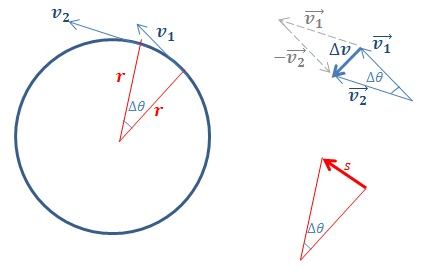# How to Find Centripetal Acceleration

Before learning how to find centripetal acceleration, let us first see what is centripetal acceleration. We will start with the definition of centripetal acceleration. The centripetal acceleration is the rate of change of tangential velocity of a body traveling in a circular path at a constant speed. Centripetal acceleration is always directed towards the centre of the circular path, and hence the name centripetal, which means “centre seeking” in Latin. In this article, we look at how to find the centripetal acceleration of an object.

## How to Derive an Expression for Centripetal Acceleration

An object moving in a circle at constant speed is accelerating. This is because acceleration involves a change in velocity. Since velocity is a vector quantity, it changes either when the magnitude of the velocity changes or when the direction of the velocity changes. Even though the object in our example is maintaining the same magnitude of velocity, the direction of velocity is changing and hence, the object is accelerating.

To find this acceleration, we consider the motion of the object during a very short time$\Delta t$. On the diagram below, the object has moved through an angle$\Delta \theta$ during the period$\Delta t$.How to find Centripetal Acceleration – Deriving Centripetal Acceleration

The velocity change during this time is given by$\triangle\vec{v}=\vec{v_{2}}-\vec{v_{1}}$. This is shown by the grey arrows in the vector triangle drawn on top right. With the blue arrows, we have placed$\vec{v_1}$ and$\vec{v_2}$ in a different arrangement to get the same$\Delta \vec{v_1}$. The reason why I have drawn the second diagram the blue vectors is because this is how the vectors are actually directed at, at the two different times considered on the diagram on left. Since the velocity vectors are always at a tangent to the circle, it then follows that the angle between the vectors$\vec{v_1}$ and$\vec{v_2}$ is also$\Delta \theta$.

Since we’re considering a very small interval of time, the distance$s$ traveled by the object during time$\Delta t$ is almost a straight line. This distance, along with the radii, is shown on the red triangle.

The blue triangle of velocity vectors and the red triangle of lengths are similar triangles. We already saw that they both contain the same angle$\Delta \theta$. Next, we realize that they are both isosceles triangles. On the red triangle, the sides attached to the angle$\Delta \theta$ are both$r$, the size of the radius.

On the blue triangle, the lengths of the sides attached to the angle$\Delta \theta$ represent the magnitudes of velocities$\vec{v_1}$ and$\vec{v_2}$. Since the object is traveling at constant velocity,$\left|\vec{v_{1}}\right|=\left|\vec{v_{2}}\right|$. This means the blue triangle is isoceles as well, and so the blue and red triangles are indeed similar.

If we take$\left|\vec{v_{1}}\right|=\left|\vec{v_{2}}\right|=v$, then we can use the similarity of triangles to say,$\frac{\Delta v}{v}=\frac{s}{r}$.

The magnitude of acceleration$a$ can be given by$\frac{\Delta v}{t}$. Then, we can write,$a=\frac{\Delta v}{t}=\frac{sv}{rt}$. Since$v=\frac{s}{r}$,$a=\frac{v^2}{r}$

Since we found$v=r \omega$ when we looked at finding angular speed, we can also write this acceleration as$a=r{\omega}^2$

We can also show that the direction of this acceleration, which is in the direction of$\vec{\Delta v}$, is directed towards the centre of the circle. Consequently, this acceleration is called centripetal acceleration because it is always pointing to the centre of the circular path.

Since the velocity of an object in circular motion is always at a tangent to the circle, this means that the acceleration is always perpendicular to the direction in which the object is moving. This is also the reason why this acceleration cannot change the magnitude of the object’s speed.

## How to Find Centripetal Acceleration

Now that we are equipped with equations, we will see how to find centripetal accelerations in different scenarios involving circular motion.

Example 1

The Earth has a radius of 6400 km. Find the centripetal acceleration on a person standing at the surface due to Earth’s rotation about its axis.How to find Centripetal Acceleration – Example 1

Example 2

A cyclist is traveling on a bicycle, which has a wheel with a radius 0.33 m. If the wheel is rotating at a constant speed, find the centripetal acceleration on a grain of sand stuck to the bicycle tyre, which is moving at a speed of 4.1 m s-1.How to find Centripetal Acceleration – Example 2

According to Newton’s second law, centripetal acceleration must be accompanied by a resultant force acting towards the centre of the circular path. This force is called the centripetal force.

## How to Calculate Centripetal Force